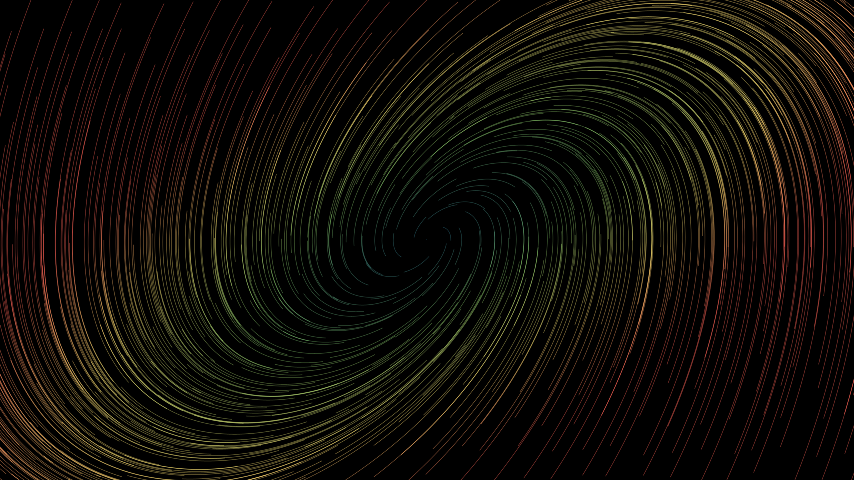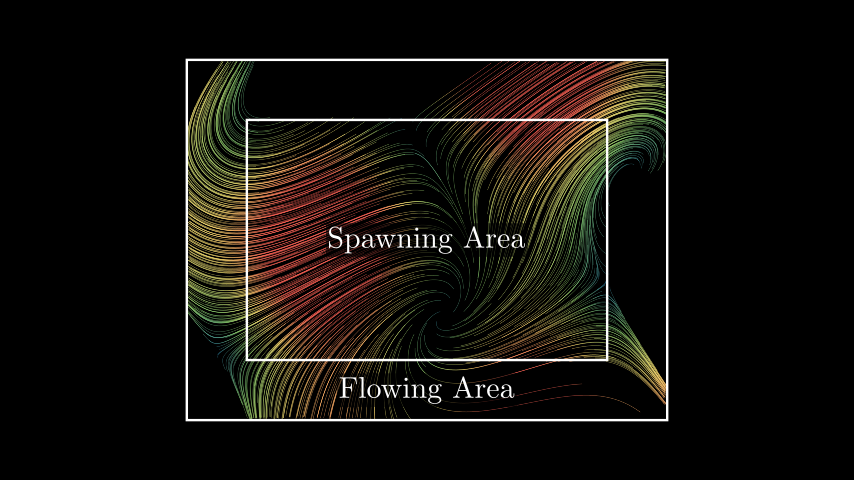# StreamLines#

Qualified name: `manim.mobject.vector\_field.StreamLines`

class StreamLines(func, color=None, color_scheme=None, min_color_scheme_value=0, max_color_scheme_value=2, colors=['#236B8E', '#83C167', '#FFFF00', '#FC6255'], x_range=None, y_range=None, z_range=None, three_dimensions=False, noise_factor=None, n_repeats=1, dt=0.05, virtual_time=3, max_anchors_per_line=100, padding=3, stroke_width=1, opacity=1, **kwargs)[source]#

Bases: `VectorField`

StreamLines represent the flow of a `VectorField` using the trace of moving agents.

Vector fields are always based on a function defining the vector at every position. The values of this functions is displayed by moving many agents along the vector field and showing their trace.

Parameters
• func (Callable[[np.ndarray], np.ndarray]) – The function defining the rate of change at every position of the vector field.

• color (Color | None) – The color of the vector field. If set, position-specific coloring is disabled.

• color_scheme (Callable[[np.ndarray], float] | None) – A function mapping a vector to a single value. This value gives the position in the color gradient defined using min_color_scheme_value, max_color_scheme_value and colors.

• min_color_scheme_value (float) – The value of the color_scheme function to be mapped to the first color in colors. Lower values also result in the first color of the gradient.

• max_color_scheme_value (float) – The value of the color_scheme function to be mapped to the last color in colors. Higher values also result in the last color of the gradient.

• colors (Sequence[Color]) – The colors defining the color gradient of the vector field.

• x_range (Sequence[float]) – A sequence of x_min, x_max, delta_x

• y_range (Sequence[float]) – A sequence of y_min, y_max, delta_y

• z_range (Sequence[float]) – A sequence of z_min, z_max, delta_z

• three_dimensions (bool) – Enables three_dimensions. Default set to False, automatically turns True if z_range is not None.

• noise_factor (float | None) – The amount by which the starting position of each agent is altered along each axis. Defaults to `delta_y / 2` if not defined.

• n_repeats – The number of agents generated at each starting point.

• dt – The factor by which the distance an agent moves per step is stretched. Lower values result in a better approximation of the trajectories in the vector field.

• virtual_time – The time the agents get to move in the vector field. Higher values therefore result in longer stream lines. However, this whole time gets simulated upon creation.

• max_anchors_per_line – The maximum number of anchors per line. Lines with more anchors get reduced in complexity, not in length.

• padding – The distance agents can move out of the generation area before being terminated.

• stroke_width – The stroke with of the stream lines.

• opacity – The opacity of the stream lines.

Examples

Example: BasicUsage```from manim import *

class BasicUsage(Scene):
def construct(self):
func = lambda pos: ((pos * UR + pos * LEFT) - pos) / 3
```

Example: SpawningAndFlowingArea```from manim import *

class SpawningAndFlowingArea(Scene):
def construct(self):
func = lambda pos: np.sin(pos) * UR + np.cos(pos) * LEFT + pos / 5
stream_lines = StreamLines(
func, x_range=[-3, 3, 0.2], y_range=[-2, 2, 0.2], padding=1
)

spawning_area = Rectangle(width=6, height=4)
flowing_area = Rectangle(width=8, height=6)
labels = [Tex("Spawning Area"), Tex("Flowing Area").shift(DOWN * 2.5)]
for lbl in labels:

```

Methods

 `create` The creation animation of the stream lines. `end_animation` End the stream line animation smoothly. `start_animation` Animates the stream lines using an updater.

Attributes

 `animate` Used to animate the application of any method of `self`. `animation_overrides` `color` `depth` The depth of the mobject. `fill_color` If there are multiple colors (for gradient) this returns the first one `height` The height of the mobject. `n_points_per_curve` `sheen_factor` `stroke_color` `width` The width of the mobject.
create(lag_ratio=None, run_time=None, **kwargs)[source]#

The creation animation of the stream lines.

The stream lines appear in random order.

Parameters
• lag_ratio (float | None) – The lag ratio of the animation. If undefined, it will be selected so that the total animation length is 1.5 times the run time of each stream line creation.

• run_time (Callable[[float], float] | None) – The run time of every single stream line creation. The runtime of the whole animation might be longer due to the lag_ratio. If undefined, the virtual time of the stream lines is used as run time.

Returns

The creation animation of the stream lines.

Return type

`AnimationGroup`

Examples

Example: StreamLineCreation

```from manim import *

class StreamLineCreation(Scene):
def construct(self):
func = lambda pos: (pos * UR + pos * LEFT) - pos
stream_lines = StreamLines(
func,
color=YELLOW,
x_range=[-7, 7, 1],
y_range=[-4, 4, 1],
stroke_width=3,
virtual_time=1,  # use shorter lines
max_anchors_per_line=5,  # better performance with fewer anchors
)
self.play(stream_lines.create())  # uses virtual_time as run_time
self.wait()
```
end_animation()[source]#

End the stream line animation smoothly.

Returns an animation resulting in fully displayed stream lines without a noticeable cut.

Returns

The animation fading out the running stream animation.

Return type

`AnimationGroup`

Raises

ValueError – if no stream line animation is running

Examples

Example: EndAnimation

```from manim import *

class EndAnimation(Scene):
def construct(self):
func = lambda pos: np.sin(pos / 2) * UR + np.cos(pos / 2) * LEFT
stream_lines = StreamLines(
func, stroke_width=3, max_anchors_per_line=5, virtual_time=1, color=BLUE
)
stream_lines.start_animation(warm_up=False, flow_speed=1.5, time_width=0.5)
self.wait(1)
self.play(stream_lines.end_animation())
```
start_animation(warm_up=True, flow_speed=1, time_width=0.3, rate_func=<function linear>, line_animation_class=<class 'manim.animation.indication.ShowPassingFlash'>, **kwargs)[source]#

Animates the stream lines using an updater.

The stream lines will continuously flow

Parameters
• warm_up (bool) – If True the animation is initialized line by line. Otherwise it starts with all lines shown.

• flow_speed (float) – At flow_speed=1 the distance the flow moves per second is equal to the magnitude of the vector field along its path. The speed value scales the speed of this flow.

• time_width (float) – The proportion of the stream line shown while being animated

• rate_func (Callable[[float], float]) – The rate function of each stream line flashing

• line_animation_class (type[ShowPassingFlash]) – The animation class being used

Return type

None

Examples

Example: ContinuousMotion

```from manim import *

class ContinuousMotion(Scene):
def construct(self):
func = lambda pos: np.sin(pos / 2) * UR + np.cos(pos / 2) * LEFT
stream_lines = StreamLines(func, stroke_width=3, max_anchors_per_line=30)